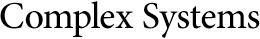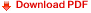## The Dynamics of a Genetic Algorithm under Stabilizing SelectionL. Magnus Rattray
Computer Science Department,
University of Manchester,
Oxford Road, Manchester M13 9PL, U.K.

#### Abstract

A formalism recently introduced [4, 5] uses the methods of statistical mechanics to model the dynamics of genetic algorithms (GAs). To be of more general interest this formalism must be able to describe problems other than the test cases considered in . In this paper, the technique is applied to the subset sum problem, which is a combinatorial optimization problem with a strongly nonlinear energy (fitness) function and many local minima under single spin flip dynamics. It is a problem that exhibits interesting dynamics, reminiscent of stabilizing selection in population biology. The dynamics are solved under certain simplifying assumptions and are reduced to a set of difference equations for a small number of relevant quantities. The quantities used are the cumulants of the population, which describe its shape, and the mean correlation within the population, which measures the microscopic similarity of population members. Including the mean correlation allows a better description of the population than the cumulants alone would provide and represents a new and important extension of the technique. The formalism includes finite population effects and describes problems of realistic size. The theory is shown to agree closely to simulations of a real GA and the mean best energy is accurately predicted.